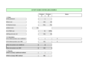•Login
•Buy credits/subscribe

# Activity Based Costing example

If your company wants to rationalise its range of products and services, you need to work out which ones to keep and which to bin. Activity Based Costing can help you to do this.

What’s Activity Based Costing?

You need to understand the cost of your company’s products and services in order to properly gauge the return made on each. Direct costs are normally easy to attribute; however, indirect costs or overheads can be more difficult. Activity Based Costing (ABC) enables you to allocate costs based on the activities consuming indirect expenses. Use our Activity Based Costing Example to help you calculate a more accurate unit cost and so identify the relative profitability of your product range.

Traditional indirect cost allocation methods divide costs across products by reference to a common denominator, for example, share of machine or labour hours. But this can result in one product subsidising others leading to incorrect decision making. ABC calculates how much of each type of indirect cost is consumed by each product.

You:

• start by identifying the resources and processes used up in the business, for example: R&D, marketing, selling and technical support
• then calculate the cost of these resources. For R&D, this could include staff, premises and the cost of any specialist materials used by that function; and
• finally for each product, estimate the usage of each type of resource, which should be directly identifiable to the product.

In our example

Our example assumes that you produce 90,000 units of product A and 10,000 of product B each year. Let’s say direct costs of labour and materials per unit are £10 for each product and that indirect costs amount to £100,000. Each product takes the same number of machine hours per unit so 90% of machine hours are consumed by product A. To simplify, we’ll assume that the indirect costs are all R&D.

Traditional method. Under traditional costing, the indirect cost of each unit of product A and product B would be £1 (£100,000/100,000 units), calculated by reference to share of machine hours. The total cost of each product would be calculated as £11 per unit (the £10 direct cost plus £1 for indirect cost allocation).

ABC method. Using ABC, we have now ascertained that it’s product B that uses all the R&D costs. So the indirect unit cost of products A and B would be £0 and £10 respectively. So under ABC, the total unit cost for products A and B would be £10 and £20 respectively.

Different results. If products A and B had been priced based on traditional costing at, say, £15 per unit, both would appear to make a £4 profit whereas using ABC, product B would actually be losing £5 per unit!

### Document10 Dec 2012
File size: 29.50K
# Pages: 2
Format:Credits: 1